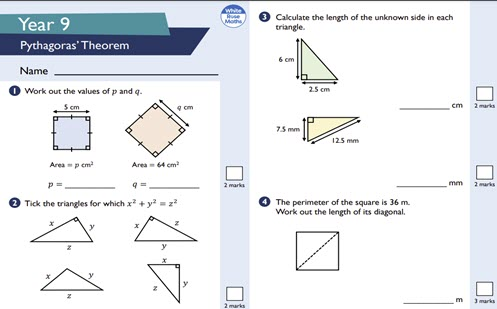# Assessment: Pythagorean Theorem

Use this two-page worksheet to assess student understanding of Pythagorean Theorem. Each question has an allocated mark.

Year level(s) Year 8
Audience Teacher
Teaching strategies and pedagogical approaches Feedback
Keywords Pythagoras Theorem

## Curriculum alignment

Strand and focus Space
Topics Pythagoras and trigonometry
AC: Mathematics (V9.0) content descriptions
AC9M8M06

Use Pythagoras' theorem to solve problems involving the side lengths of right-angled triangles

Numeracy progression Understanding geometric properties (P7)
Understanding units of measurement (P10)
Multiplicative strategies (P9)